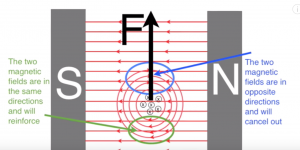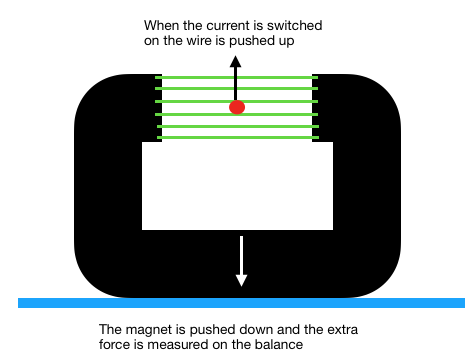### Measuring magnetic flux density

When a wire or a coil of wires is carrying a current there will be a magnetic field around it, like this:Force on a coil in a magnetic field

If that coil is then placed in another large magnetic field, then the two fields interact imposing a force on the wire and logically (using Newtons Third Law) an equal and opposite force on the other magnet. Looking at a side view of the magnet the magnetic field below the wire is reinforced whereas the two fields above the wire oppose each other so the overall field is weak. The wire is then pushed up towards the weaker field and the magnet is pushed down.

In the video below these facts are used to measure the force in an experimental set up like this:Experimental setup to measure the force on a current carrying wire

The force depends on the strength of the field, the number of wires in the coil, the length of the coil in the magnet and on the current flowing through the coil. In this setup the force F=NBIL where F is the force in newtons, N is the number of turns in the coil, I is the current, L the length of the coil with the magnetic field and B the magnetic flux density.

We rearrange that to find the flux density of the field of the large magnet, so B = F/NIL

There is a PDF of notes to download which extends the examples of forces in a magnetic field

Forces in a magnetic field

### Measuring magnetic flux density video lesson

Other useful pages on magnetism and electromagnetism  are: